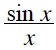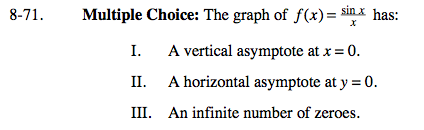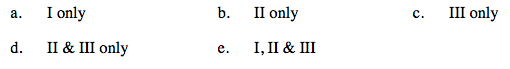### Home > CALC > Chapter 8 > Lesson 8.2.1 > Problem8-71

8-71.
1. Multiple Choice: The graph of f(x) =has: Homework Help ✎

1. A vertical asymptote at x = 0.

2. A horizontal asymptote at y = 0.

3. An infinite number of zeroes.

1. I only

2. II only

3. III only

4. II & III only

5. I, II & III$\text{Check: }\lim_{x\rightarrow 0-}f(x)= \ \ \text{ and } \ \ \lim_{x\rightarrow 0+}f(x)=$

Check: f(x) = 0